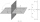# Line + combinatorial number - math problems

#### Number of problems found: 5

• LinesHow many lines can be draw with 8 points, if three points lie on one line and the other any three points do not lie on the same line?
• CirclesHow many different circles is determined by 9 points at the plane, if 6 of them lie in a straight line?
• Points in planeThe plane is given 12 points, 5 of which is located on a straight line. How many different lines could by draw from this points?
• Points in spaceThere are n points, of which no three lie on one line and no four lies on one plane. How many planes can be guided by these points? How many planes are there if there are five times more than the given points?
• LinesIn how many points will intersect 14 different lines, where no two are parallel?

We apologize, but in this category are not a lot of examples.
Do you have an interesting mathematical word problem that you can't solve it? Submit a math problem, and we can try to solve it.

We will send a solution to your e-mail address. Solved examples are also published here. Please enter the e-mail correctly and check whether you don't have a full mailbox.

Please do not submit problems from current active competitions such as Mathematical Olympiad, correspondence seminars etc...

See also our combinations calculator. Line - math word problems. Combinatorial number - math word problems.